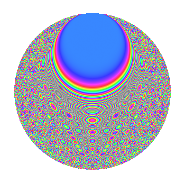# Properties

 Label 287.2.bbLevel 287 Weight 2 Character orbit bb Rep. character $$\chi_{287}(6,\cdot)$$ Character field $$\Q(\zeta_{40})$$ Dimension 416 Newforms 1 Sturm bound 56 Trace bound 0

# Related objects

## Defining parameters

 Level: $$N$$ = $$287 = 7 \cdot 41$$ Weight: $$k$$ = $$2$$ Character orbit: $$[\chi]$$ = 287.bb (of order $$40$$ and degree $$16$$) Character conductor: $$\operatorname{cond}(\chi)$$ = $$287$$ Character field: $$\Q(\zeta_{40})$$ Newforms: $$1$$ Sturm bound: $$56$$ Trace bound: $$0$$

## Dimensions

The following table gives the dimensions of various subspaces of $$M_{2}(287, [\chi])$$.

Total New Old
Modular forms 480 480 0
Cusp forms 416 416 0
Eisenstein series 64 64 0

## Trace form

 $$416q - 32q^{2} - 40q^{4} - 16q^{7} - 48q^{8} - 48q^{9} + O(q^{10})$$ $$416q - 32q^{2} - 40q^{4} - 16q^{7} - 48q^{8} - 48q^{9} - 32q^{11} - 12q^{14} - 8q^{15} + 56q^{16} - 24q^{18} + 4q^{21} - 64q^{22} - 40q^{23} - 40q^{25} - 32q^{28} - 24q^{29} - 8q^{30} + 32q^{32} - 16q^{35} - 96q^{36} + 48q^{37} - 32q^{39} - 192q^{42} - 8q^{43} + 128q^{44} + 48q^{46} - 48q^{49} - 120q^{50} + 48q^{51} - 32q^{53} - 124q^{56} - 8q^{57} + 56q^{58} - 152q^{60} + 112q^{63} - 40q^{64} - 120q^{65} - 96q^{67} + 32q^{70} + 64q^{71} - 40q^{72} - 72q^{74} + 76q^{77} + 128q^{78} - 40q^{79} + 304q^{84} - 48q^{85} - 40q^{86} + 24q^{88} + 132q^{91} - 144q^{92} + 24q^{93} - 32q^{95} + 88q^{98} - 48q^{99} + O(q^{100})$$

## Decomposition of $$S_{2}^{\mathrm{new}}(287, [\chi])$$ into irreducible Hecke orbits

Label Dim. $$A$$ Field CM Traces $q$-expansion
$$a_2$$ $$a_3$$ $$a_5$$ $$a_7$$
287.2.bb.a $$416$$ $$2.292$$ None $$-32$$ $$0$$ $$0$$ $$-16$$